We all know that in Python we can for loop to iterate through a list, tuple or range object. So what is the underlying principle?

In understanding Python’s data structures, containers (container), iterable objects (iterator), iterators (iterator), generators (generator), lists/sets/dictionary comprehensions (list, set, dict comprehension), and many other concepts are mixed together to make beginners confused. The relationship between them.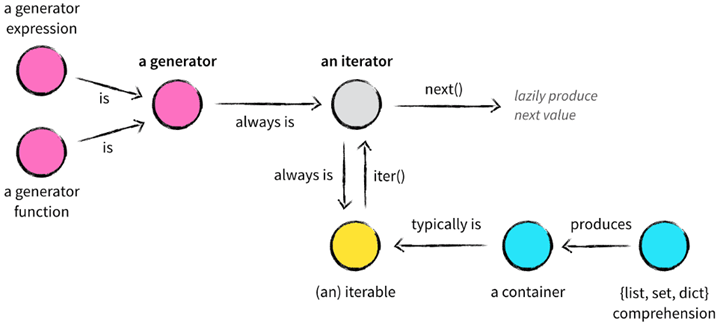## container

A container is a data structure that organizes multiple elements together. The elements in a container can be obtained iteratively one by one, and you can use the in, not in keywords to determine whether an element is contained in the container. Usually this type of data structure stores all elements in memory (there are some exceptions where not all elements are in memory, such as iterator and generator objects) In Python, common container objects are.

• list, deque, ….
• set, frozensets, ….
• dict, defaultdict, OrderedDict, Counter, ….
• tuple, namedtuple, …
• str

From a technical point of view, when it can be used to ask whether an element is contained in it, then the object can be considered a container, for example, lists, collections, and tuples are all container objects. Generally containers are iterable objects, but not all containers are iterable.

## iterable

For any object in Python that defines a `__iter__` method that returns an iterator, or a `__getitem__` method that supports subscript indexing, the object is iterable. In Python, all containers, such as lists, tuples, dictionaries, collections, etc., are iterable.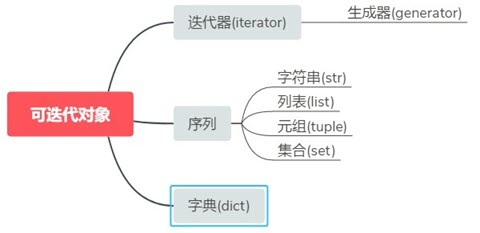Method for determining whether an object is iterable.

 `````` 1 2 3 4 5 6 7 8 9 10 11 12 13 14 15 16 17 18 19 20 21 22 23 24 25 26 27 28 29 30 31 `````` ``````from collections.abc import Iterable def iterable_test_1(obj): return isinstance(obj, Iterable) def iterable_test_2(obj): try: iter(obj) return True except TypeError: return False def iterable_test_3(obj): try: for i in obj: pass return True except TypeError: return False if __name__ == "__main__": print(iterable_test_1("Hello World!")) print(iterable_test_2("Hello World!")) print(iterable_test_3("Hello World!")) print(iterable_test_1(123)) print(iterable_test_2(123)) print(iterable_test_3(123)) ``````

In practical applications it is recommended to use isinstance() to make the determination.

## iterator

Iterators refer to tools that iterate over values; iteration is an iterative process; each iteration is based on the result of the previous one; iterators provide a generic and index-independent way of iterating over values.

Iterators should implement not only the `__iter__` method, but also the `__next__` method.

• `__iter__`: returns the iterator itself self.
• `__next__`: returns the next available element of the iterator, throwing a StopIteration exception when there is no element at the end.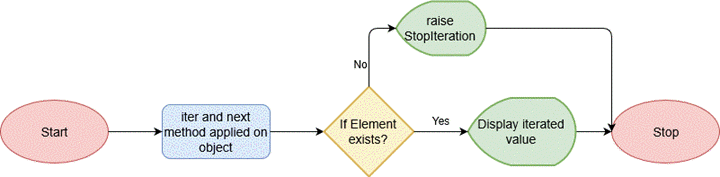Characteristics of iterators.

• An iterator must be an iterable object because the `__iter__` method is implemented.
• The `__iter__` method of an iterator returns itself, does not produce a new iterator object, and can only be iterated over once. If you want to iterate again you need to rebuild the iterator.
• Iterators are inert computations that return values only when called, and wait for the next call when not called. This saves a lot of memory space.

Iterator protocol: The object must provide a next method, the execution of which either returns the next item in the iteration or raises a StopIteration exception to terminate the iteration (it can only go backwards not forwards).

Pros.

• Provides a generic and index-independent way of iterating over values
• Only one value exists in memory at the same time, which is more memory-efficient

• Not as flexible as indexed fetching (can’t fetch a specified value and can only go backwards)
• Unable to predict the length of the iterator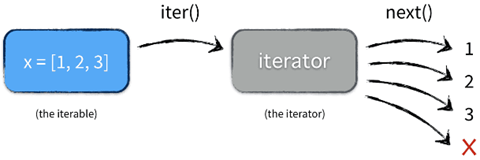`````` 1 2 3 4 5 6 7 8 9 10 11 12 13 14 15 16 17 18 19 20 21 22 23 24 25 26 27 `````` ``````# 创建一个迭代器类 class Fib: def __init__(self): self.prev = 0 self.curr = 1 def __iter__(self): return self def __next__(self): value = self.curr self.curr += self.prev self.prev = value if value > 500: raise StopIteration return value print(Fib()) # 根据可迭代对象创建一个迭代器 lst = [1, 2, 3] res = iter(lst) print(res) # 判断可迭代对象是否为迭代器 from collections.abc import Iterator print(isinstance(lst, Iterator)) print(isinstance(res, Iterator)) ``````

## generator

A generator is both iterable and iterative, and there are two ways to define a generator.

• generator function
• generator expression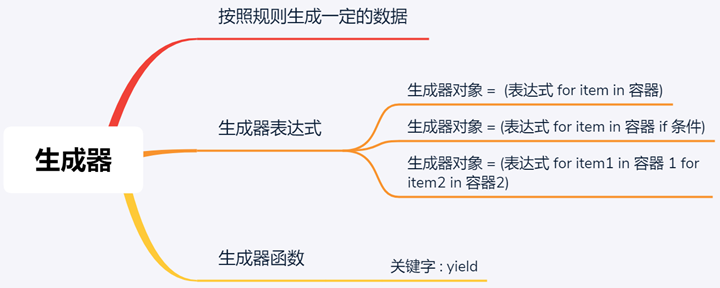### generator function

If a function definition contains the yield keyword, the entire function is a generator function. During the execution of a generator function, each time it encounters a yield, the function pauses and saves all the current run information, returns the value of the yield, and continues from the current position the next time the next() method is executed.

 `````` 1 2 3 4 5 6 7 8 9 10 11 12 13 14 `````` ``````def fibonacci(n): a, b, counter = 1, 1, 0 while counter < n: yield a a, b = b, a + b counter += 1 f = fibonacci(10) print(next(f)) print(next(f)) print('----') for item in f: print(item) ``````

Generator functions look and behave like regular functions, but have one defining feature. Instead of return, generator functions use the Python yield keyword. yield indicates where to send the value back to the caller, and unlike return, you don’t exit the function afterwards. Instead, it remembers the state of the function. Thus, when next() is called on a generator object (explicitly or implicitly in a for loop), the value is generated again.

### generator expression

A generator expression is a generator version of a list pushdown that looks like a list derivative, but it returns a generator object instead of a list object. A generator expression is computed on demand (or inert), computing values when needed and returning an iterator, while a list parser returns values immediately, returning a list of iterable objects. In terms of memory footprint, generator expressions are more memory-efficient.

 ``````1 2 3 4 5 6 7 8 9 `````` ``````nums_squared_lc = [num**2 for num in range(5)] nums_squared_gc = (num**2 for num in range(5)) if __name__ == "__main__": print(nums_squared_lc) print(nums_squared_gc) # 输出 # [0, 1, 4, 9, 16] # at 0x0000022D4FD5EDC8> ``````

### Example of generator usage

#### Example 1: Reading large files

A common use case for generators is working with data streams or large files, such as CSV files. Now, what if you want to count the number of rows in a CSV file? The following code gives some ideas.

 ``````1 2 3 4 5 6 7 `````` ``````csv_gen = csv_reader("some_csv.txt") row_count = 0 for row in csv_gen: row_count += 1 print(f"Row count is {row_count}") ``````

You may want csv_gen to be a list. To populate this list, csv_reader() opens a file and loads its contents into csv_gen. The program then iterates through the list and increments row_count for each line.

This is a reasonable logic, but does this design still work if the file is very large? What if the file is larger than the available memory? Let’s assume for a moment that csv_reader() simply opens the file and reads it into an array.

 ``````1 2 3 4 `````` ``````def csv_reader(file_name): file = open(file_name) result = file.read().split("\n") return result ``````

This function opens a given file and adds each line as a separate element to the list using file.read(), with.split(). When executed you may get the following output.

 ``````1 2 3 4 5 6 7 8 `````` ``````Traceback (most recent call last): File "ex1_naive.py", line 22, in main() File "ex1_naive.py", line 13, in main csv_gen = csv_reader("file.txt") File "ex1_naive.py", line 6, in csv_reader result = file.read().split("\n") MemoryError ``````

In this case, open() returns a generator object and you can iterate over it lazily line by line. However, file.read().split() loads all the contents into memory at once, leading to MemoryError. so, how to handle these huge data files? Look at redefining csv_reader().

 ``````1 2 3 `````` ``````def csv_reader(file_name): for row in open(file_name, "r"): yield row ``````

In this version, you open the file, iterate over it, and produce a single line. This code should produce the following output, with no memory errors. What’s happening here? You’ve actually turned csv_reader() into a generator function. Open a file, iterate over each line, and produce each line instead of returning it.

You can also define generator expressions whose syntax is very similar to list derivatives. This makes it possible to use generators without calling the function.

 ``````1 `````` ``````csv_gen = (row for row in open(file_name)) ``````

#### Example 2: Generating an infinite sequence

To get a finite sequence in Python, you range() can be called in the context of a list and evaluate it.

 ``````1 2 `````` ``````a = range(5) print(list(a)) ``````

However, generating an infinite sequence requires the use of a generator, since your computer’s memory is limited.

 `````` 1 2 3 4 5 6 7 8 9 10 `````` ``````def infinite_sequence(): num = 0 while True: yield num num += 1 if __name__ == "__main__": for i in infinite_sequence(): print(i, end=" ") ``````

The program will execute consistently until you stop it manually. In addition to using a for loop, you can also next() to call the generator object directly. This is particularly useful for testing generators in the console: the

 ``````1 2 `````` ``````gen = infinite_sequence() print(next(gen)) ``````

#### Example 3: Back to text detection

You can use infinite sequences in many ways, but one practical use for them is to construct an echo detector. An echo detector will find all letters or sequences that are an echo. That is, forward and backward to be able to read the same word or number, for example 121. First, define your numeric echo detector.

 `````` 1 2 3 4 5 6 7 8 9 10 11 12 13 14 15 16 17 18 19 20 21 22 23 24 25 26 27 28 29 `````` ``````def infinite_sequence(): num = 0 while True: yield num num += 1 def is_palindrome(num): # Skip single-digit inputs if num // 10 == 0: return False temp = num reversed_num = 0 while temp != 0: reversed_num = (reversed_num * 10) + (temp % 10) temp = temp // 10 if num == reversed_num: return num else: return False if __name__ == "__main__": for i in infinite_sequence(): pal = is_palindrome(i) if pal: print(i) ``````

Don’t worry too much about understanding the underlying math in this code. This function takes an input number, inverts it, and checks to see if the inverted number is the same as the original number. The only numbers printed to the console are those that are the same forward or backward.

### generator performance

You learned earlier that generators are a great way to optimize memory. While the infinite sequence generator is an extreme example of this optimization, let’s zoom in on the number-squared example you just saw and examine the size of the resulting object. You can do this by calling sys.getsizeof().

 `````` 1 2 3 4 5 6 7 8 9 10 `````` ``````nums_squared_lc = [num**2 for num in range(10000)] nums_squared_gc = (num**2 for num in range(10000)) if __name__ == "__main__": import sys print(sys.getsizeof(nums_squared_lc)) print(sys.getsizeof(nums_squared_gc)) # 输出 # 87624 # 120 ``````

In this case, you get a list of 87624 bytes from the list derivation, while the generator object has only 120. This means that the list is more than 700 times larger than the generator object! However, there is one thing to remember. If the list is smaller than the available memory of the running machine, then the list derivation will compute faster than the equivalent generator expression:

 `````` 1 2 3 4 5 6 7 8 9 10 11 12 13 14 15 16 17 18 19 20 21 22 23 24 25 26 27 28 29 30 `````` ``````import cProfile print(cProfile.run('sum([i * 2 for i in range(10000)])')) print(cProfile.run('sum((i * 2 for i in range(10000)))')) # 输出 # 5 function calls in 0.001 seconds # # Ordered by: standard name # # ncalls tottime percall cumtime percall filename:lineno(function) # 1 0.001 0.001 0.001 0.001 :1() # 1 0.000 0.000 0.001 0.001 :1() # 1 0.000 0.000 0.001 0.001 {built-in method builtins.exec} # 1 0.000 0.000 0.000 0.000 {built-in method builtins.sum} # 1 0.000 0.000 0.000 0.000 {method 'disable' of '_lsprof.Profiler' objects} # # # None # 10005 function calls in 0.002 seconds # # Ordered by: standard name # # ncalls tottime percall cumtime percall filename:lineno(function) # 10001 0.001 0.000 0.001 0.000 :1() # 1 0.000 0.000 0.002 0.002 :1() # 1 0.000 0.000 0.002 0.002 {built-in method builtins.exec} # 1 0.001 0.001 0.002 0.002 {built-in method builtins.sum} # 1 0.000 0.000 0.000 0.000 {method 'disable' of '_lsprof.Profiler' objects} # # # None ``````

You have seen the most common uses and constructs of generators, but there are a few more tricks to introduce. In addition to yield, the following methods are available for generator objects.

• .send()
• .throw()
• .close()

#### How to use .send()

 `````` 1 2 3 4 5 6 7 8 9 10 11 12 13 14 15 16 17 18 19 20 21 22 23 24 25 26 27 28 29 30 31 32 33 `````` ``````def infinite_palindromes(): num = 0 while True: if is_palindrome(num): i = (yield num) if i is not None: num = i num += 1 def is_palindrome(num): # Skip single-digit inputs if num // 10 == 0: return False temp = num reversed_num = 0 while temp != 0: reversed_num = (reversed_num * 10) + (temp % 10) temp = temp // 10 if num == reversed_num: return True else: return False if __name__ == "__main__": pal_gen = infinite_palindromes() for i in pal_gen: digits = len(str(i)) pal_gen.send(10 ** (digits)) print(i) ``````

This program will print numeric palindromes as before, but with a few tweaks. When a palindrome is encountered, your new program will add a number and start searching for the next one from there.

• infinite_palindromes() in i = (yield num), check if i is not None, which may happen if next() is called on a generator object.
• send(), will execute 10 ** digits to i, update num

What you are creating here is a coroutine (concatenation), or a generator function that can pass data. These are useful for building data pipelines.

#### How to use .throw()

.throw() allows you to use generators to throw exceptions. In the following example, this code will throw a ValueError when digits=5 is thrown.

 ``````1 2 3 4 5 6 7 8 9 `````` ``````if __name__ == "__main__": pal_gen = infinite_palindromes() for i in pal_gen: print(i) digits = len(str(i)) if digits == 5: pal_gen.throw(ValueError("We don't like large palindromes")) pal_gen.send(10 ** (digits)) print(i) ``````

.throw() is useful in any area where you may need to catch an exception.

#### How to use .close()

As the name implies, .close() allows you to stop the generator. This is particularly handy when controlling an infinite sequence generator.

 ``````1 2 3 4 5 6 7 8 9 `````` ``````if __name__ == "__main__": pal_gen = infinite_palindromes() for i in pal_gen: print(i) digits = len(str(i)) if digits == 5: pal_gen.close() pal_gen.send(10 ** (digits)) print(i) ``````

.close() is that it raises StopIteration an exception to indicate the end of the finite iterator.

### Creating data pipelines using generators

 ``````1 2 3 4 5 6 7 8 `````` ``````file_name = "techcrunch.csv" lines = (line for line in open(file_name)) list_line = (s.rstrip().split(",") for s in lines) cols = next(list_line) company_dicts = (dict(zip(cols, data)) for data in list_line) funding = (int(company_dict["raisedAmt"]) for company_dict in company_dicts if company_dict["round"] == "a") total_series_a = sum(funding) print(f"Total series A fundraising: \${total_series_a}") ``````

## for loop working mechanism

After introducing iterable objects and iterators, let’s further summarize the working mechanism of for loops in iterable objects and iterators.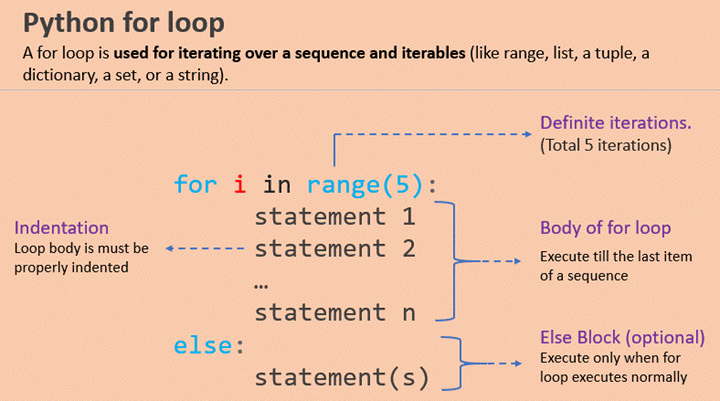Iterable objects in the for loop work mechanism.

• first determine whether the object is an iterable object (equivalent to determining whether there is an iter or getitem method), if not iterable then throw a TypeError exception. If the object is iterable then the `__iter__` method is called and an iterator is returned.
• Keep calling the `__next__` method of the iterator, returning one value from the iterator at a time, in order.
• Throws an exception StopIteration when there are no elements at the end of the iteration. this exception is handled by Python itself and is not exposed to the developer.

Iterator in for loop working mechanism.

• Call the `__iter__` method, which returns its own self, i.e., the iterator.
• Keep calling the iterator’s next() method, returning one value from the iterator at a time, in order.
• Throws an exception StopIteration when there are no elements at the end of the iteration.

In Python, for loops are compatible with two mechanisms.

• If the object defines `__iter__`, an iterator is returned.
• If the object does not define `__iter__` but implements `__getitem__`, it will iterate over subscripts instead.

When the for loop finds no `__iter__` but `__getitem__`, it will read the corresponding subscripts in order, starting from 0 until an IndexError occurs. iter() method also handles this case, returning a subscripted iterator object instead when `__iter__` is not present.

## Generator functions in the standard library

Generator functions for filtering.

Module Function Description
itertools compress(it, selector_it) Processes two iterable objects in parallel. If the element in selector_it is true, output the corresponding element in it
itertools dropwhile(predicate, it) Passes the elements of the iterable object it to predicate, skips elements where predicate(item) is true, stops when predicate(item) is false, and outputs all remaining (unskipped) elements (no further checking)
Built-in filter(predicate, it) Pass each element of it to predicate, and if predicate(item) returns true, output the corresponding element
itertools filterfalse(predicate, it) Similar to the filter function, but predicate(item) returns the corresponding element when it returns a false value
itertools takewhile(predicate, it) predicate(item) produces the corresponding element when it returns the true value, and then stops immediately without further checking
itertools islice(it, stop) or islice(it, start, stop, step=1) produces a slice of it, similar to s[:stop] or s[start:stop:step, except that it can be any iterable object and implements an inert operation

Generator functions for mapping.

Module Function Description
itertools accumulate(it, [func]) The cumulative sum of the outputs. If func is provided, the first two elements of it are passed to func, then the result of the calculation is passed to func along with the next element, and so on, yielding the result
Built-in enumerate(it, start=0) yields a tuple of two elements, with the structure (index, item). where index is counted from start and item is obtained from it
Built-in map(func, it1, [it2, …, itN]) Pass each element of it to func to produce the result; if you pass in N iterables, func must be able to accept N arguments and process each iterable in parallel

Generator function for merging multiple iterable objects.

Module Function Description
itertools chain(it1, …, itN) Output all the elements in it1, then all the elements in it2, and so on, seamlessly
itertools chain.from_iterable(it) Produce the elements of each iterable object generated by it, one after the other seamlessly; the elements in it should be iterable objects (i.e. it is an iterable object with nested iterables)
itertools product(it1, …, itN, repeat=1) Computes the Cartesian product. Takes the elements from each of the input iterables and combines them into a tuple of N elements, the same effect as a nested for loop. repeat specifies how many times to repeat the input iterables
Built-in zip(it1, …, itN) The elements are fetched from each of the input iterable objects in parallel, resulting in a tuple of N elements. As soon as any of these iterable objects reaches the end, it simply stops
itertools zip_longest(it1, …, itN, fillvalue=None) The elements are fetched from each of the input iterable objects in parallel, producing a tuple of N elements, and stopping only when the longest iterable object is reached. The missing values are filled with fillvalue

Generator functions for rearranging elements.

Module Function Description
itertools groupby(it, key=None) Produces an element consisting of two elements of the form (key, group), where key is the grouping criterion and group is the generator used to produce the elements in the group
Built-in reversed(seq) Output the elements of seq in reverse order, from back to front; seq must be a sequence or an object that implements the `__reversed__` special method
itertools tee(it, n=2) produces a tuple of n generators, each of which can independently produce the elements of the input iterable object

Functions that read an iterator and return a single value.

Module Function Description
Built-in all(it) True if all elements in it are true, False otherwise; all([]) returns True
Built-in any(it) True if it has an element that is true, otherwise False; any([]) returns False
Built-in max(it, [key=], [default=]) Returns the element with the largest value in it; key is the sorting function, the same as in sorted; if the iterable object is empty, returns default
Built-in min(it, [key=], [default=]) Returns the element with the smallest value in it; key is the sorting function; if the iterable object is empty, returns default
functools reduce(func, it, [initial]) Pass the first two elements to func, then pass the result of the computation and the third element to func, and so on, returning the final result. If initial is provided, pass it as the first element into
Built-in sum(it, start=0) The sum of all elements in it, and if an optional start is provided, it will be added as well